# PROBABILITY

Probability is a way of expressing knowledge or belief that an event will occur or has occurred. The concept has an exact mathematical meaning in probability theory, which is used extensively in such areas of study as mathematics, statistics, finance, gambling, science, artificial intelligence/machine learning and philosophy to draw conclusions about the likelihood of potential events and the underlying mechanics of complex systems.

## Interpretations

The word probability does not have a consistent direct definition. In fact, there are two broad categories of probability interpretations, whose adherents possess different (and sometimes conflicting) views about the fundamental nature of probability:

1. Frequentists talk about probabilities only when dealing with experiments that are random and well-defined. The probability of a random event denotes the relative frequency of occurrence of an experiment’s outcome, when repeating the experiment. Frequentists consider probability to be the relative frequency “in the long run” of outcomes.
2. Bayesians, however, assign probabilities to any statement whatsoever, even when no random process is involved. Probability, for a Bayesian, is a way to represent an individual’s degree of belief in a statement, or an objective degree of rational belief, given the evidence.

## Etymology

The word Probability derives from the Latin probabilitas, which can also mean probity, a measure of the authority of a witness in a legal case in Europe, and often correlated with the witness’s nobility. In a sense, this differs much from the modern meaning of probability, which, in contrast, is a measure of the weight of empirical evidence, and is arrived at from inductive reasoning and statistical inference.

## History

The scientific study of probability is a modern development. Gambling shows that there has been an interest in quantifying the ideas of probability for millennia, but exact mathematical descriptions arose much later. There are reasons of course, for the slow development of the mathematics of probability. Whereas games of chance provided the impetus for the mathematical study of probability, fundamental issues are still obscured by the superstitions of gamblers.

According to Richard Jeffrey, “Before the middle of the seventeenth century, the term ‘probable’ (Latin probabilis) meant approvable, and was applied in that sense, univocally, to opinion and to action. A probable action or opinion was one such as sensible people would undertake or hold, in the circumstances.” However, in legal contexts especially, ‘probable’ could also apply to propositions for which there was good evidence.

Aside from elementary work by Girolamo Cardano in the 16th century, the doctrine of probabilities dates to the correspondence of Pierre de Fermat and Blaise Pascal (1654). Christiaan Huygens (1657) gave the earliest known scientific treatment of the subject. Jakob Bernoulli’s Ars Conjectandi (posthumous, 1713) and Abraham de Moivre’s Doctrine of Chances (1718) treated the subject as a branch of mathematics. See Ian Hacking’s The Emergence of Probability and James Franklin’s The Science of Conjecture for histories of the early development of the very concept of mathematical probability.

The theory of errors may be traced back to Roger Cotes’s Opera Miscellanea (posthumous, 1722), but a memoir prepared by Thomas Simpson in 1755 (printed 1756) first applied the theory to the discussion of errors of observation. The reprint (1757) of this memoir lays down the axioms that positive and negative errors are equally probable, and that certain assignable limits define the range of all errors. Simpson also discusses continuous errors and describes a probability curve.

Pierre-Simon Laplace (1774) first tried to deduce a rule for combining observations from the principles of the theory of probabilities. He represented the law of probability of errors by a curve y = φ(x), x being any error and y its probability, and laid down three properties of this curve:

1. It is symmetric as to the y-axis;
2. The x-axis is an asymptote, the probability of the error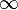being 0;
3. The area enclosed is 1, it being certain that an error exists.

He also provided, in 1781, a formula for the law of facility of error (a term Lagrange used in 1774), but it led to unmanageable equations. Daniel Bernoulli (1778) introduced the principle of the maximum product of the probabilities of a system of concurrent errors.

Adrien-Marie Legendre (1805) developed the method of least squares, and introduced it in his Nouvelles méthodes pour la détermination des orbites des comètes (New Methods for Determining the Orbits of Comets). In ignorance of Legendre’s contribution, an Irish-American writer, Robert Adrain, editor of “The Analyst” (1808), first deduced the law of facility of error,h being a constant depending on precision of observation, and c a scale factor ensuring that the area under the curve equals 1. He gave two proofs, the second being essentially the same as John Herschel’s (1850). Gauss gave the first proof that seems to have been known in Europe (the third after Adrain’s) in 1809. Further proofs were given by Laplace (1810, 1812), Gauss (1823), James Ivory (1825, 1826), Hagen (1837), Friedrich Bessel (1838), W. F. Donkin (1844, 1856), and Morgan Crofton (1870). Other contributors were Ellis (1844), De Morgan (1864), Glaisher (1872), and Giovanni Schiaparelli (1875). Peters’s (1856) formula for r, the probable error of a single observation, is well known.

In the nineteenth century authors on the general theory included Laplace, Sylvestre Lacroix (1816), Littrow (1833), Adolphe Quetelet (1853), Richard Dedekind (1860), Helmert (1872), Hermann Laurent (1873), Liagre, Didion, and Karl Pearson. Augustus De Morgan and George Boole improved the exposition of the theory.

Andrey Markov introduced the notion of Markov chains (1906), which played an important role in stochastic processes theory and its applications. The modern theory of probability based on the measure theory was developed by Andrey Kolmogorov (1931).

On the geometric side (see integral geometry) contributors to The Educational Times were influential (Miller, Crofton, McColl, Wolstenholme, Watson, and Artemas Martin).

Further information: History of probability
Further information: History of statistics

## Theory

Main article: Probability theory

Like other theories, the theory of probability is a representation of probabilistic concepts in formal terms—that is, in terms that can be considered separately from their meaning. These formal terms are manipulated by the rules of mathematics and logic, and any results are interpreted or translated back into the problem domain.

There have been at least two successful attempts to formalize probability, namely the Kolmogorov formulation and the Cox formulation. In Kolmogorov’s formulation (see probability space), sets are interpreted as events and probability itself as a measure on a class of sets. In Cox’s theorem, probability is taken as a primitive (that is, not further analyzed) and the emphasis is on constructing a consistent assignment of probability values to propositions. In both cases, the laws of probability are the same, except for technical details.

There are other methods for quantifying uncertainty, such as the Dempster-Shafer theory or possibility theory, but those are essentially different and not compatible with the laws of probability as usually understood.

## Applications

Probability theory is applied in everyday life in risk assessment and in trade on commodity markets. Governments typically apply probabilistic methods in environmental regulation, where it is called pathway analysis. A good example is the effect of the perceived probability of any widespread Middle East conflict on oil prices—which have ripple effects in the economy as a whole. An assessment by a commodity trader that a war is more likely vs. less likely sends prices up or down, and signals other traders of that opinion. Accordingly, the probabilities are not assessed independently nor necessarily very rationally. The theory of behavioral finance emerged to describe the effect of such groupthink on pricing, on policy, and on peace and conflict.

It can reasonably be said that the discovery of rigorous methods to assess and combine probability assessments has profoundly affected modern society. Accordingly, it may be of some importance to most citizens to understand how odds and probability assessments are made, and how they contribute to reputations and to decisions, especially in a democracy.

Another significant application of probability theory in everyday life is reliability. Many consumer products, such as automobiles and consumer electronics, use reliability theory in product design to reduce the probability of failure. Failure probability may influence a manufacture’s decisions on a product’s warranty.

## Mathematical treatment

Consider an experiment that can produce a number of results. The collection of all results is called the sample space of the experiment. The power set of the sample space is formed by considering all different collections of possible results. For example, rolling a die can produce six possible results. One collection of possible results give an odd number on the die. Thus, the subset {1,3,5} is an element of the power set of the sample space of die rolls. These collections are called “events.” In this case, {1,3,5} is the event that the die falls on some odd number. If the results that actually occur fall in a given event, the event is said to have occurred.

A probability is a way of assigning every event a value between zero and one, with the requirement that the event made up of all possible results. (In our example, the event {1,2,3,4,5,6}) is assigned a value of one.) To qualify as a probability, the assignment of values must satisfy the requirement that if you look at a collection of mutually exclusive events (events with no common results, e.g., the events {1,6}, {3}, and {2,4} are all mutually exclusive), the probability that at least one of the events will occur is given by the sum of the probabilities of all the individual events.

The probability of an event A is written as P(A), p(A) or Pr(A). This mathematical definition of probability can extend to infinite sample spaces, and even uncountable sample spaces, using the concept of a measure.

The opposite or complement of an event A is the event [not A] (that is, the event of A not occurring); its probability is given by P(not A) = 1 – P(A). As an example, the chance of not rolling a six on a six-sided die is 1 – (chance of rolling a six)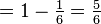. See Complementary event for a more complete treatment.

If both events A and B occur on a single performance of an experiment, this is called the intersection or joint probability of A and B, denoted as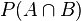. If two events, A and B are independent then the joint probability is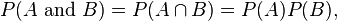for example, if two coins are flipped the chance of both being heads is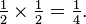If either event A or event B or both events occur on a single performance of an experiment this is called the union of the events A and B denoted as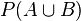. If two events are mutually exclusive then the probability of either occurring isFor example, the chance of rolling a 1 or 2 on a six-sided die isIf the events are not mutually exclusive thenFor example, when drawing a single card at random from a regular deck of cards, the chance of getting a heart or a face card (J,Q,K) (or one that is both) is, because of the 52 cards of a deck 13 are hearts, 12 are face cards, and 3 are both: here the possibilities included in the “3 that are both” are included in each of the “13 hearts” and the “12 face cards” but should only be counted once.

Conditional probability is the probability of some event A, given the occurrence of some other event B. Conditional probability is written P(A|B), and is read “the probability of A, given B“. It is defined by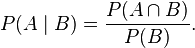If P(B) = 0 thenis undefined. Note that in this case A and B are independent.

Summary of probabilities
Event Probability
A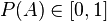not A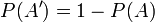A or BA and BA given B## Relation to randomness

In a deterministic universe, based on Newtonian concepts, there would be no probability if all conditions are known, (Laplace’s demon). In the case of a roulette wheel, if the force of the hand and the period of that force are known, the number on which the ball will stop would be a certainty. Of course, this also assumes knowledge of inertia and friction of the wheel, weight, smoothness and roundness of the ball, variations in hand speed during the turning and so forth. A probabilistic description can thus be more useful than Newtonian mechanics for analyzing the pattern of outcomes of repeated rolls of roulette wheel. Physicists face the same situation in kinetic theory of gases, where the system, while deterministic in principle, is so complex (with the number of molecules typically the order of magnitude of Avogadro constant 6.02·1023) that only statistical description of its properties is feasible.

Probability theory is required to describe nature. A revolutionary discovery of early 20th century physics was the random character of all physical processes that occur at sub-atomic scales and are governed by the laws of quantum mechanics. The objective wave function evolves deterministically but, according to the Copenhagen interpretation, randomness is explained by a wave function collapse when an observation is made. However, the loss of determinism for the sake of instrumentalism did not meet with universal approval. Albert Einstein famously remarked in a letter to Max Born: “I am convinced that God does not play dice”. Like Einstein, Erwin Schrödinger, who discovered the wave function, believed quantum mechanics is a statistical approximation of an underlying deterministic reality. In modern interpretations, quantum decoherence accounts for subjectively probabilistic behavior.Welcome to my blog. My name is Nico. Admin of this blog. I am a student majoring in mathematics who dreams of becoming a professor of mathematics. I live in Kwadungan, Ngawi, East Java. Hopefully in all the posts I can make a good learning material to the intellectual life of the nation. After the read, leave a comment. I always accept criticism suggestion to build a better me again .. Thanks for visiting .. : mrgreen:

Posted on July 1, 2011, in education and tagged , , . Bookmark the permalink. 9 Comments.

1.dymo

wow

2.ageng

Dari kalimat pertama saya udah manggut-manggut
Sampe kalimat terakhir, saya nangis-nangis T.T

•elnicovengeance

g perlu nangis-nangis…. hehehehe

3.veera

heheh. tumben posting englishbetewe, ngubah font-nya ini gimana sih kak?

•elnicovengeance

gpp.. lagi pengen aja..
aq pake typekit font.. kunjungi aja http://www.typekit.com

4.cold4hands
•elnicovengeance